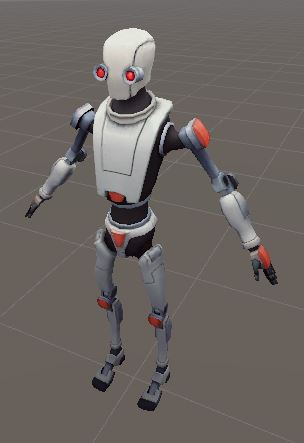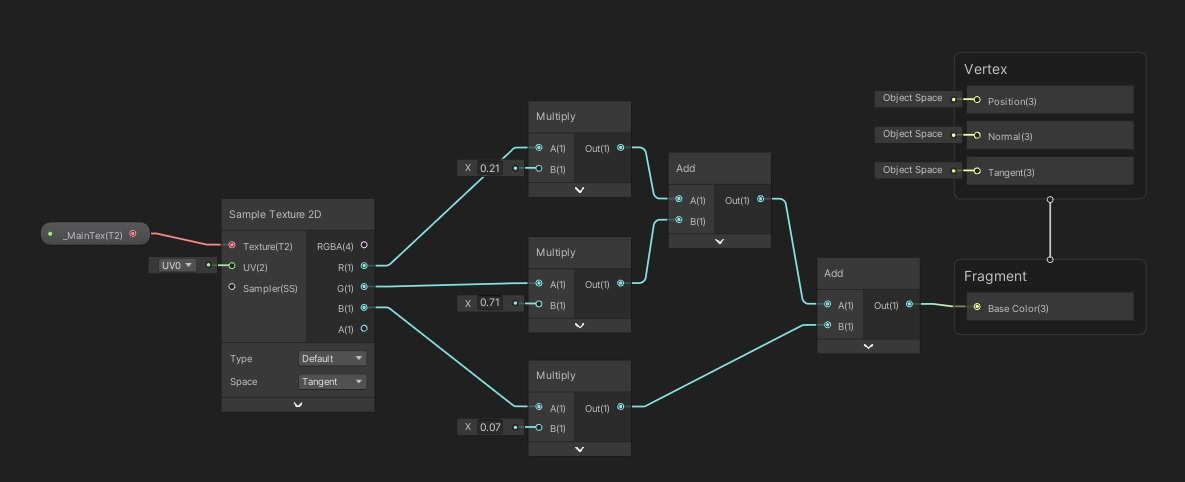• Out = (Red + Green + Blue) / 3
• Out = (Red * 0.3 + Green * 0.59 + Blue * 0.11) 或 Out = (Red * 0.2126 + Green * 0.7152 + Blue * 0.0722) 或 Out = (Red * 0.299 + Green * 0.587 + Blue * 0.114)
• Out = ( Math.max(Red, Green, Blue) + Math.min(Red, Green, Blue) ) / 2
• Out = Math.max(Red, Green, Blue) 或 Out = Math.min(Red, Green, Blue)
• Out = Red 或 Out = Green 或 Out = Blue

ConversionFactor = 255 / (NumberOfShades - 1)
AverageValue = (Red + Green + Blue) / 3
Gray = Math.round((AverageValue / ConversionFactor) + 0.5) * ConversionFactor